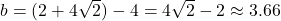## A positive real number is 4 less than another. If the sum of the squares of the two numbers is 72, then find the numbers.

Question

A positive real number is 4 less than another. If the sum of the squares of the two numbers is 72, then find the numbers.

in progress 0
5 months 2021-08-15T00:42:02+00:00 2 Answers 27 views 0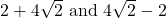Step-by-step explanation:

Let the large number be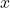. We can represent the smaller number with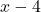. Since their squares add up to 72, we have the following equation: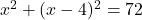Expand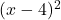using the property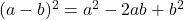: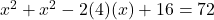Combine like terms: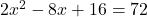Subtract 72 from both sides: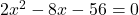Use the quadratic formula to find solutions for: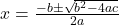for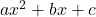In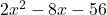, assign:

•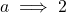•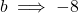•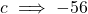Solving, we get: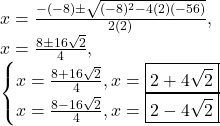Since the question stipulates thatis positive, we have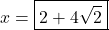. Therefore, the two numbers are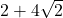and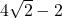.

Verify: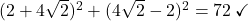Our two numbers are: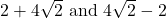Or, approximately 7.66 and 3.66.

Step-by-step explanation:

Let the two numbers be a and b.

One positive real number is four less than another. So, we can write that: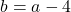The sum of the squares of the two numbers is 72. Therefore: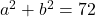Substitute: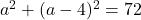Solve for a. Expand: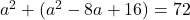Simplify: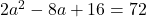Divide both sides by two: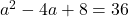Subtract 36 from both sides: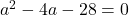The equation isn’t factorable. So, we can use the quadratic formula: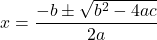In this case, a = 1, b = -4, and c = -28. Substitute: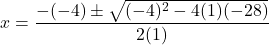Evaluate: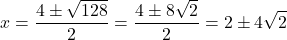So, our two solutions are: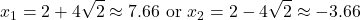Since the two numbers are positive, we can ignore the second solution.

So, our first number is: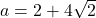And since the second number is four less, our second number is: